Solve this simultaneous equation x+2y=11 x-2y=1

Question

Solve this simultaneous equation x+2y=11
x-2y=1

in progress 0
5 months 2021-08-27T12:13:35+00:00 2 Answers 0 views 0

using elimination method multiply both equations by 2…then subtract equation 2 from 1

Equation 1,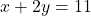Equation 2,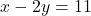Take equation number 2.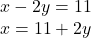Substitute ‘x’ in Equation number 1.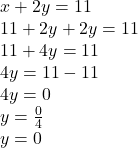y = 0.

So x,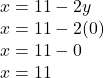x = 11.